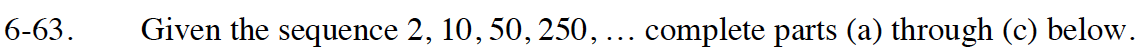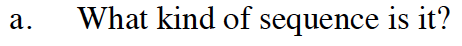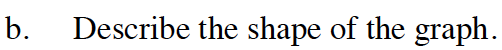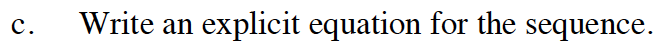Home > GB8I > Chapter cc46 > Lesson cc46.2.2 > Problem6-63

6-63.
1. Given the sequence 2, 10, 50, 250, … complete parts (a) through (c) below. Homework Help ✎

1. What kind of sequence is it?

2. Describe the shape of the graph.

3. Write an explicit equation for the sequence.How do you get from one term to the next?The shape of the graph is curved.What is t(0)? What is the multiplier?

$t(n)=\frac{2}{5}(5)^{n}$

Make sure you know why this is the answer.